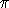CGAL::Iso_rectangle_2<Kernel>

Definition

An object s of the data type Iso_rectangle_2<Kernel> is a rectangle in the Euclidean plane2 with sides parallel to the x and y axis of the coordinate system.

Although they are represented in a canonical form by only two vertices, namely the lower left and the upper right vertex, we provide functions for ``accessing'' the other vertices as well. The vertices are returned in counterclockwise order.

Iso-oriented rectangles and bounding boxes are quite similar. The difference however is that bounding boxes have always double coordinates, whereas the coordinate type of an iso-oriented rectangle is chosen by the user.

Creation

Iso_rectangle_2<Kernel> r ( Point_2<Kernel> p, Point_2<Kernel> q);
introduces an iso-oriented rectangle r with diagonal opposite vertices p and q. Note that the object is brought in the canonical form.

 Iso_rectangle_2 r ( Point_2 left, Point_2 right, Point_2 bottom, Point_2 top);
introduces an iso-oriented rectangle r whose minimal x coordinate is the one of left, the maximal x coordinate is the one of right, the minimal y coordinate is the one of bottom, the maximal y coordinate is the one of top.

 Iso_rectangle_2 r ( Kernel::RT min_hx, Kernel::RT min_hy, Kernel::RT max_hx, Kernel::RT max_hy, Kernel::RT hw = RT(1));
introduces an iso-oriented rectangle r with diagonal opposite vertices (min_hx/hw, min_hy/hw) and (max_hx/hw, max_hy/hw).
Precondition: hw0.

Operations

 bool r.operator== ( r2) Test for equality: two iso-oriented rectangles are equal, iff their lower left and their upper right vertices are equal. bool r.operator!= ( r2) Test for inequality. Point_2 r.vertex ( int i) returns the i'th vertex modulo 4 of r in counterclockwise order, starting with the lower left vertex. Point_2 r.operator[] ( int i) returns vertex(i). Point_2 r.min () returns the lower left vertex of r (= vertex(0)). Point_2 r.max () returns the upper right vertex of r (= vertex(2)). Kernel::FT r.xmin () returns the x coordinate of lower left vertex of r. Kernel::FT r.ymin () returns the y coordinate of lower left vertex of r. Kernel::FT r.xmax () returns the x coordinate of upper right vertex of r. Kernel::FT r.ymax () returns the y coordinate of upper right vertex of r. Kernel::FT r.min_coord ( int i) returns the i'th Cartesian coordinate of the lower left vertex of r. Precondition: 0i1. Kernel::FT r.max_coord ( int i) returns the i'th Cartesian coordinate of the upper right vertex of r. Precondition: 0i1.

Predicates

 bool r.is_degenerate () r is degenerate, if all vertices are collinear. Bounded_side r.bounded_side ( Point_2 p) returns either ON_UNBOUNDED_SIDE, ON_BOUNDED_SIDE, or the constant ON_BOUNDARY, depending on where point p is. bool r.has_on_boundary ( Point_2 p) bool r.has_on_bounded_side ( Point_2 p) bool r.has_on_unbounded_side ( Point_2 p)

Miscellaneous

 Kernel::FT r.area () returns the area of r. Bbox r.bbox () returns a bounding box containing r. Iso_rectangle_2 r.transform ( Aff_transformation_2 t) returns the iso-oriented rectangle obtained by applying t on the lower left and the upper right corner of r. Precondition: The angle at a rotation must be a multiple of/2, otherwise the resulting rectangle does not have the same side length. Note that rotating about an arbitrary angle can even result in a degenerate iso-oriented rectangle.